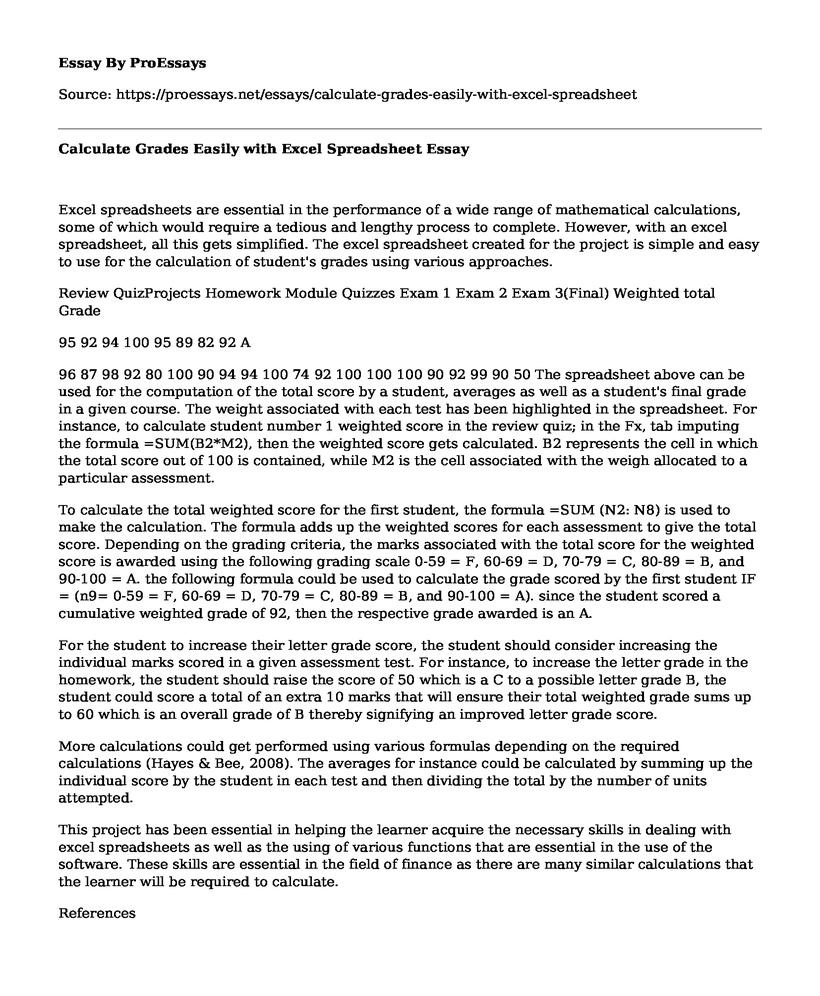Paper Type:  Essay Pages:  2 Wordcount:  547 Words Date:  2023-02-23
Categories:

Excel spreadsheets are essential in the performance of a wide range of mathematical calculations, some of which would require a tedious and lengthy process to complete. However, with an excel spreadsheet, all this gets simplified. The excel spreadsheet created for the project is simple and easy to use for the calculation of student's grades using various approaches.

Is your time best spent reading someone else’s essay? Get a 100% original essay FROM A CERTIFIED WRITER!

Review QuizProjects Homework Module Quizzes Exam 1 Exam 2 Exam 3(Final) Weighted total Grade

95 92 94 100 95 89 82 92 A

96 87 98 92 80 100 90 94 94 100 74 92 100 100 100 90 92 99 90 50 The spreadsheet above can be used for the computation of the total score by a student, averages as well as a student's final grade in a given course. The weight associated with each test has been highlighted in the spreadsheet. For instance, to calculate student number 1 weighted score in the review quiz; in the Fx, tab imputing the formula =SUM(B2*M2), then the weighted score gets calculated. B2 represents the cell in which the total score out of 100 is contained, while M2 is the cell associated with the weigh allocated to a particular assessment.

To calculate the total weighted score for the first student, the formula =SUM (N2: N8) is used to make the calculation. The formula adds up the weighted scores for each assessment to give the total score. Depending on the grading criteria, the marks associated with the total score for the weighted score is awarded using the following grading scale 0-59 = F, 60-69 = D, 70-79 = C, 80-89 = B, and 90-100 = A. the following formula could be used to calculate the grade scored by the first student IF = (n9= 0-59 = F, 60-69 = D, 70-79 = C, 80-89 = B, and 90-100 = A). since the student scored a cumulative weighted grade of 92, then the respective grade awarded is an A.

For the student to increase their letter grade score, the student should consider increasing the individual marks scored in a given assessment test. For instance, to increase the letter grade in the homework, the student should raise the score of 50 which is a C to a possible letter grade B, the student could score a total of an extra 10 marks that will ensure their total weighted grade sums up to 60 which is an overall grade of B thereby signifying an improved letter grade score.

More calculations could get performed using various formulas depending on the required calculations (Hayes & Bee, 2008). The averages for instance could be calculated by summing up the individual score by the student in each test and then dividing the total by the number of units attempted.

This project has been essential in helping the learner acquire the necessary skills in dealing with excel spreadsheets as well as the using of various functions that are essential in the use of the software. These skills are essential in the field of finance as there are many similar calculations that the learner will be required to calculate.

References

Hayes, D. C., & Bee, S. (2008). Powering up your Grade book: A Spreadsheet Designed to Teach Students Excel Skills and to Make Assigning Students' Grades Easier. AIS Educator Journal, 3(1), 15-33.Free essays can be submitted by anyone,

so we do not vouch for their quality

Want a quality guarantee?
Order from one of our vetted writers instead

If you are the original author of this essay and no longer wish to have it published on the ProEssays website, please click below to request its removal:

Liked this essay sample but need an original one?

Hire a professional with VAST experience!